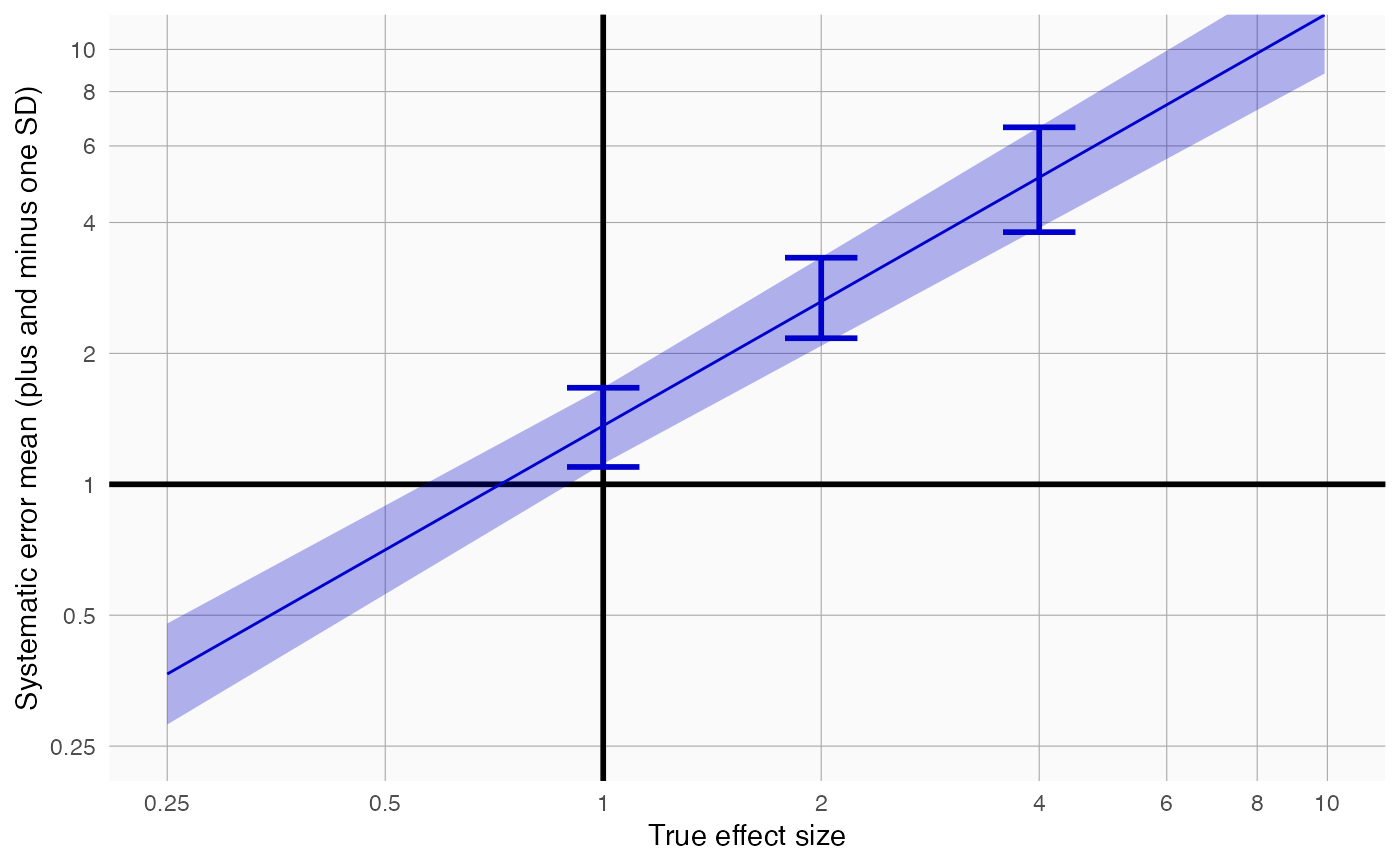plotErrorModel creates a plot showing the systematic error model.

plotErrorModel(
logRr,
seLogRr,
trueLogRr,
title,
legacy = FALSE,
fileName = NULL
)

## Arguments

logRr

A numeric vector of effect estimates on the log scale.

seLogRr

The standard error of the log of the effect estimates. Hint: often the standard error = (log(<lower bound 95 percent confidence interval>) - log(<effect estimate>))/qnorm(0.025).

trueLogRr

The true log relative risk.

title

Optional: the main title for the plot

legacy

If true, a legacy error model will be fitted, meaning standard deviation is linear on the log scale. If false, standard deviation is assumed to be simply linear.

fileName

Name of the file where the plot should be saved, for example 'plot.png'. See the function ggsave in the ggplot2 package for supported file formats.

## Value

A Ggplot object. Use the ggsave function to save to file.

## Details

Creates a plot with the true effect size on the x-axis, and the mean plus and minus the standard deviation shown on the y-axis. Also shown are simple error models fitted at each true relative risk in the input.

## Examples

data <- simulateControls(n = 50 * 3, mean = 0.25, sd = 0.25, trueLogRr = log(c(1, 2, 4)))
plotErrorModel(data$logRr, data$seLogRr, data\$trueLogRr)